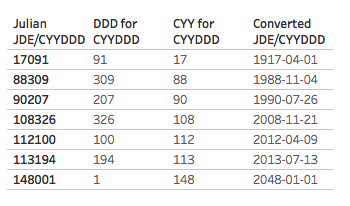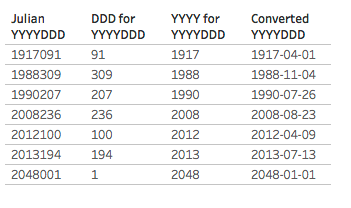# Tag Archives: JDE# A Dating Lesson: Using Math to Get Dates (by Converting Julian Dates)

I recently had to convert some date fields formatted as Julian dates into more usable Tableau date data types (it’s not a date format that Tableau natively understands) and my web search got me a little frustrated because all the solutions I found were long formulas using string manipulation functions like LEFT(), RIGHT(), etc. That kind of solution can get the job done, but there’s something to be left desired:

String manipulation is sloooooooooooooooooooooooooooooowwww.

How slow? How about one thousand times slower (or more) than using math functions! I like my calculations to be efficient from the beginning: The practical reason is that I don’t have to spend time later going back to do performance tuning. Computers were built to do math really really fast so whenever possible I’ll use math to do my date conversions. The aesthetic reason is that there’s an elegance to creating equations that use the least number of steps, and the play reason is because math is fun!

Therefore in this post I’ll describe a faster, more elegant, and more fun way (IMO) of converting Julian dates into Tableau dates. Note that in some cases you might be able to do the conversion to a date data type inside your database, that could be even faster than the formulas I describe here.

## What is a Julian date, anyways?

There are two formats of Julian dates that I’ve run into:

• The JDE (for JD Edwards) or CYYDDD format where the DDD is the day of the year (a number from 1 to 366), YY is the two digit year, and C is the century where 0 is 1900, 1 is 2000, and so on. For example 5001 is 1905-01-01 and 117365 is 2017-12-31.
• The “modern Julian date” or YYYYDDD format where DDD is again the day of year and YYYY is the four digit year, so 2017365 would be 2017-12-31.

Now the Julian dates might be stored in raw data as strings or as numbers, either way we’re going to work with them as numbers. So if you’re starting out with Julian dates as strings then the first step is to right-click on those fields and change the data type to Number (Whole).

## Learning Three Math & Date Techniques

We can convert Julian JDE/CYYDDD dates in a single formula:

```DATE(DATEADD('day',[Julian JDE/CYYDDD] % 1000 - 1,

There are three techniques at work in this formula:

1. Extract the right-most N digits from a number using the modulo operator, for example to get the DDD value.
2. Remove the right-most N digits from a number to extract the left-most M digits from a number using the INT() function, for example to get the CYY value.
3. Using DATEADD() to add intervals to a starting date and nest for each date_part as necessary.

### Extracting the right-most N digits from a number

The slow way to get the right-most N digits from a number would be something like INT(RIGHT(STR([number]),N)). We can completely avoid the string conversion and need for RIGHT() by using some math.

The modulo operator % is a math function that returns the remainder for a whole number. So 5 % 2 returns 1, 17 % 10 returns 7, and so on. We can use this to return the right-most N digits from a number without needing to do any string manipulation by using [number] % 10^N.

In our case `[Julian JDE/CYYDDD] % 1000` will return the last 3 digits of the field corresponding to the DDD aka day of year.

A couple of other uses of the modulo function are jittering marks in a dot plot and creating groups/bins based on the right-most digits when numbers are categorical variables.

### Remove the right-most N digits from a number to extract the left-most M digits from the number

The starting way to get the left-most M digits from a number would be something like INT(LEFT(STR([number]),M)). However this gets more complicated with the JDE/CYYDDD format because depending on the date then we might want the 2 left most digits (for years in the 1900s) or the 3 left most digits (for years in the 2000s) which would lead to even more string manipulation to get the desired result, for example here’s a formula I found online for getting the year: `IF LEN([Julian JDE/CYYDDD]) = 6 AND LEFT([Julian JDE/CYYDDD], 1) = '1' THEN '20' + MID([Julian JDE/CYYDDD], 2, 2) ELSEIF LEN([Julian JDE/CYYDDD]) = 5 THEN '19' + MID([Julian JDE/CYYDDD], 1, 2) END`. All those LEN(), LEFT(), and MID() calls will be slooooww.

Thinking about this from a math standpoint what we want to do is with the JDE/CYYDDD format is to remove the DDD (the right-most N digits) and extract what’s left. The way we can do that is in two steps: 1) turn the DDD portion into a decimal so CYYDDD becomes CYY.DDD, then 2) truncate the DDD decimal portion so we’re just left with the CYY.

We can do that with the formula INT([number] / 10^N). The [number] / 10^N divides the number by the number of digits we want to remove, so 5001 becomes 5.001, 117365 becomes 117.365, and so on. Then the INT() around that truncates the decimal places and leaves us with the remaining whole number.

In our case` INT([Julian JDE/CYYDDD] / 1000)` removes the right-most three digits so what we’re left with is the century and number of years.

I regularly use this technique to build my own bins, for example INT([Sales] / 100) * 100 creates bins of 0, 100, 200, and so on.

### Using DATEADD() to add a number of intervals to a starting date

Tableau’s DATEADD() function takes three arguments:

• date_part: a value like ‘day’, ‘month’, ‘hour’, etc.
• interval: a whole number of intervals
• date: a starting date

The advantage of using DATEADD() over something like DATE([lots of string manipulation to build a string of YYYY-MM-DD or MM/DD/YYYY or DD/MM/YYYY format]) is again that we’re avoiding all that string manipulation and just doing date math, which is really math.

With the first two techniques we’ve converted the JDE/CYYDDD format to into the intervals for the day date_part and the intervals for the year date_part, so now we can use DATEADD() once for each date_part in a nested fashion. All we need is a starting date, and in this case we can take advantage of the CYY structure to figure out the appropriate starting date. This is the number of years since 1900, so to generate the year we can use:

`DATEADD('year',INT([Julian JDE/CYYDDD]/1000),#1900-01-01#)`

Then to add the DDD day of year we can use DATEADD() with a starting date of the year date we just made. We do need to subtract 1 from the DDD day of year because the first day of the year is 1, not 0.

```DATEADD('day',[Julian JDE/CYYDDD] % 1000 - 1,

## Julian JDE/CYYDDD Conversion

Tableau’s DATEADD() always returns a datetime data type, so using the above formula and wrapping the calculation in a final DATE() gives us the desired date data type:

```DATE(DATEADD('day',[Julian JDE/CYYDDD] % 1000 - 1,

Here’s a Tableau view showing the DDD and CYY calculations along with the final Converted JDE/CYYYDDD calculation:## Julian YYYYDDD Conversion

The YYYYDDD conversion uses a quite similar formula, with one change:

```DATE(DATEADD('day',[Julian YYYYDDD] % 1000 - 1,

The difference is that the YYYY portion of the calculation is returning the full four digit year so we subtract 1900 from that to get a number of years to add to the 1900-01-01 start date.

Here’s a Tableau view showing the DDD and YYYY calculations along with the final Converted YYYYDDD calculation:## Conclusion

Math is fun. Math is fast. Math is your friend. Use math to get dates. (Date data types, that is).

Here’s a link to the converting julian dates to dates workbook on Tableau Public.

A little plug: If you like this post (and can tolerate the occasional bad pun) and want help in finding dates (in your data) please check out my company, DataBlick. We offer Tableau and Alteryx support, consulting, and training and can help you get the most out of your data!